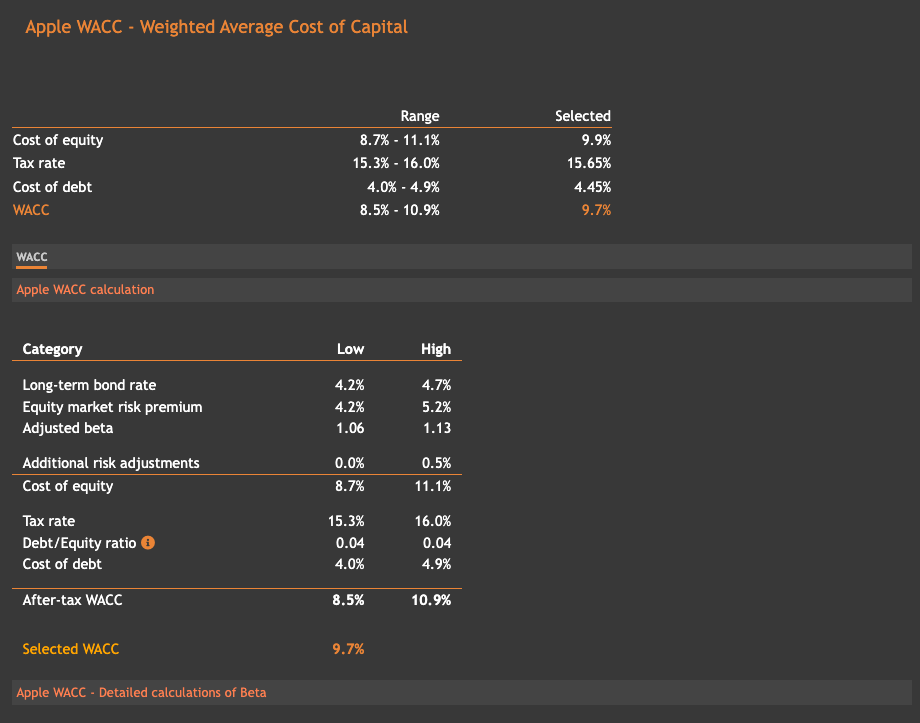# WACC Calculator

Accurate and science-based method to instantly compute Weighted Average Cost of Capital (WACC).
Our calculator contains the pre-calculated WACC for all global companies.

What is Weighted Average Cost of Capital (WACC)?

WACC is a company's average after-tax cost of capital.

WACC depends heavily on a company's capital structure. Most companies have a mix of capital sources such as common stocks, preferred stocks, debts, etc... WACC is simply the weighted average of those sources' cost.

Many refer to WACC as the expected rate the company has to pay to finance its operations. WACC is the key component of all DCF models and is used to discount future cash flows to the present day

Some components of WACC, such as the effective interest rate and tax rate, have objective values and can be obtained easily using the financial statements. Some other components can only be calculated in a subjective manner such as equity risk-premium and beta. In these cases, we usually rely on historical data and estimates of experts such as Professor Damodaran's estimates of equity risk-premium for all markets globally.How is WACC calculated?

There are multiple components needed to compute a company's WACC.

WACC = (E / V) × CE + (D / V) × CD × (1 - T)

where

• E -- Market capitalization
• D -- Market value of the company's debt
• V -- E + D, total market value of debt and equity financing
• CD -- Cost of debt
• CE -- Cost of equity
• T -- Tax rate

If you would like to better understand the 2 core elements of WACC and how they are calculated, you can check out cost of equity calculator and cost of debt calculator. They go into more details about how each smaller component like risk-free rate, tax rate and market risk-premium affect the final WACC.

Sample WACCs